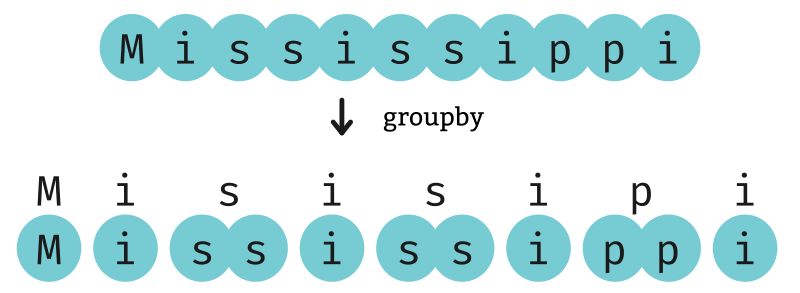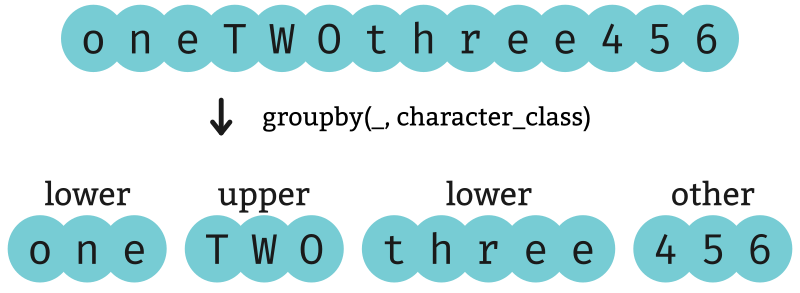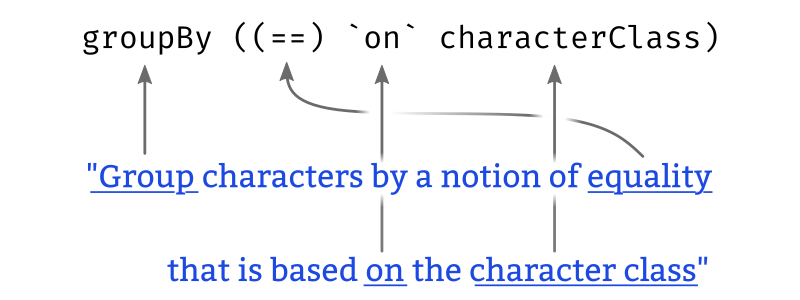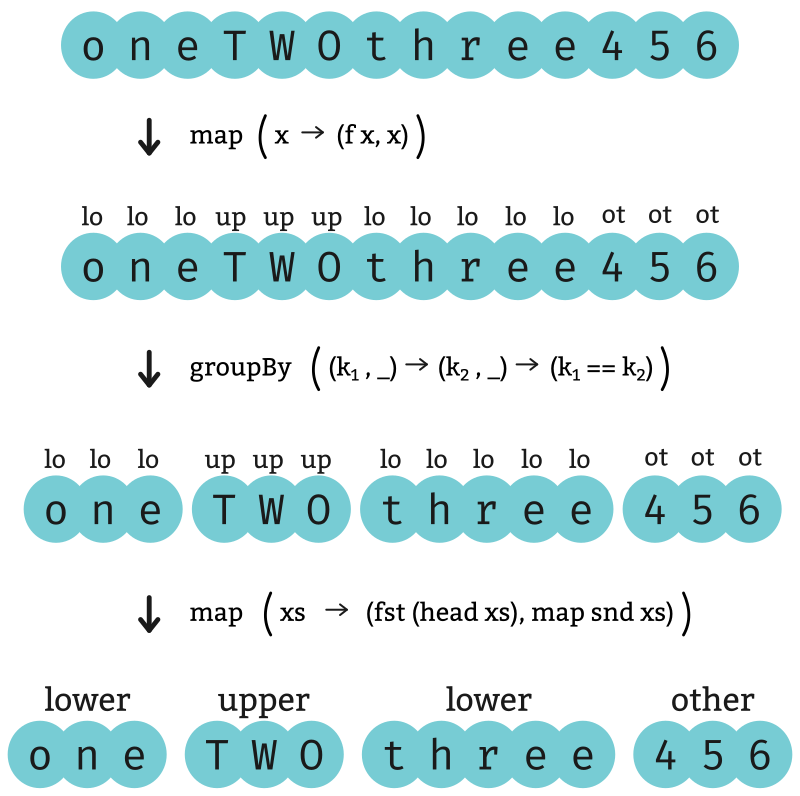# Grouping

The itertools `groupby` function divides a sequence into contiguous subsequences where the elements in each subsequence share something in common.

## Grouping by equality

When used with a single parameter, `groupby` finds groups of repeated elements. The reason this function is named “groupby” instead of “group” won’t become clear until we discuss the optional `key` parameter.Each output of the resulting list is a tuple of two components: A representative element of the group (e.g. `'s'`) and the group itself (e.g. `['s', 's']`).

``````>>> list(k for k, g in itertools.groupby('Mississippi'))
['M', 'i', 's', 'i', 's', 'i', 'p', 'i']``````
``````>>> list(list(g) for k, g in itertools.groupby('Mississippi'))
[['M'], ['i'], ['s', 's'], ['i'], ['s', 's'], ['i'], ['p', 'p'], ['i']]``````

Haskell’s `group` function `group` in `Data.List` produces a list of the groups.

``````λ> group "Mississippi"
["M","i","ss","i","ss","i","pp","i"]``````

Its output does not also include a representative element of the group as Python’s does, but if this is desired then it can be recovered by taking the `head` of each group. `head` in `Data.List` and `Prelude`.

``````λ> map head (group "Mississippi")
"Misisipi"``````

This is a situation where composing the two functions

1. `map head`
2. `group`

using the function composition operator, `(.)`, might read more nicely.

``````λ> (map head . group) "Mississippi"
"Misisipi"``````

## The `key` parameter

Let’s say we have a function that categorizes characters into one of three varieties:

1. lower-case;
2. upper-case; or
3. “other”.
``````def character_class(x):
if x.islower(): return 'lower'
if x.isupper(): return 'upper'
return 'other'``````

If we use this `character_class` function as the `key` for `groupby`, then the grouping is now performed not based on whether elements are the same, but based on whether elements belong to the same character class.

``````>>> it = itertools.groupby('oneTWOthree456', character_class)

>>> list((k, ''.join(g)) for k, g in it)
[('lower', 'one'), ('upper', 'TWO'), ('lower', 'three'), ('other', '456')]``````

The first element of each tuple (`k` in the example above) now tells us which class the characters in the group belong to. The second element gives the characters themselves.Now again in Haskell! First we’ll define an equivalent of the `character_class` function using Haskell’s guard syntax: `isLower` and `isUpper` in `Data.Char`

``````characterClass x
| isUpper x = "upper"
| isLower x = "lower"
| otherwise = "other"``````

We can use the `groupBy` function for this. `groupBy` in `Data.List` Its first argument serves a purpose similar to that of the `key` parameter, but instead of a unary function that produces a key, we need to give it a binary function that determines whether two elements belong to the same group.

We want two elements to end up in the same group if they have the same character class, so the function we need to use is

``\x y -> characterClass x == characterClass y``

So our grouping then looks like this:

``````λ> groupBy (\x y -> characterClass x == characterClass y) "oneTWOthree456"
["one","TWO","three","456"]``````

We can write this a bit more concisely using the `on` function: `on` in `Data.Function`

``````λ> groupBy ((==) `on` characterClass) "oneTWOthree456"
["one","TWO","three","456"]``````

The purpose of the `on` function is to be used exactly like this, and the idea behind its name is that the expression reads a bit like an English phrase:This does not, however, give us the value of the key for each group like the Python function did; it only does the grouping, it doesn’t tell us which character class each group belongs to. If we want that, we have to write our own function. The implementation we give below uses three steps:

``````groupByKey f
= map (\xs -> (fst (head xs), map snd xs))  -- 3
. groupBy ((==) `on` fst)                   -- 2
. map (\x -> (f x, x))                      -- 1``````
``````λ> groupByKey characterClass "oneTWOthree456"
[("lower","one"),("upper","TWO"),("lower","three"),("other","456")]``````
1. Apply the key function to each input value, keeping both the key and the value
2. Use `groupBy` to group the list according to the key
3. For each group, produce the a tuple containing:
• `fst (head xs)`: The key of the first item in the group; (It doesn’t matter which item we look at, since the group values all have the same key)
• `map snd xs`: The values in the group.Join Type Classes for courses and projects to get you started and make you an expert in FP with Haskell.